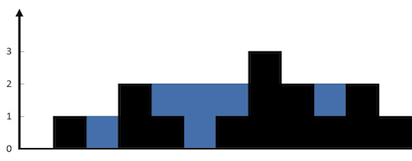# 42 Trapping Rain Water

### Problem:

Given n non-negative integers representing an elevation map where the width of each bar is 1, compute how much water it is able to trap after raining.

For example, Given [0,1,0,2,1,0,1,3,2,1,2,1], return 6.The above elevation map is represented by array [0,1,0,2,1,0,1,3,2,1,2,1]. In this case, 6 units of rain water (blue section) are being trapped. Thanks Marcos for contributing this image!

### Solutions:

``````public class Solution {
public int trap(int[] height) {
int left = 0;
int leftIndex = -1;
int water = 0;
int inside = 0;
HashSet<String> range = new HashSet<String>();
for (int i = 0; i < height.length; i ++) {
if (height[i] >= left) {
water = water + left * (i - leftIndex - 1) - inside;
left = height[i];
leftIndex = i;
inside = 0;

}
else {
inside += height[i];
}
}
int right = 0;
int rightIndex = height.length;
inside = 0;
for (int i = height.length - 1; i >= 0; i --) {
if (height[i] >= right) {
if (!range.contains(i+","+rightIndex)) {
water = water + right * (rightIndex - i - 1) - inside;
}
right = height[i];
rightIndex = i;
inside = 0;
}
else {
inside += height[i];
}
}
return water;
}
}
``````
``````public class Solution {
public int trap(int[] height) {
if (height.length == 0) {
return 0;
}
int[] left = new int[height.length];
int[] right = new int[height.length];
int max = height;
left = height;
for (int i = 1; i < height.length; i ++) {
if (height[i] > max) {
max = height[i];
}
left[i] = max;
}
max = height[height.length - 1];
right[height.length - 1] = height[height.length - 1];
for (int i = height.length - 2; i >= 0; i --) {
if (height[i] > max) {
max = height[i];
}
right[i] = max;
}
int water = 0;
for (int i = 0; i < height.length; i ++) {
water +=Math.min(left[i], right[i]) - height[i];
}
return water;
}
}
``````